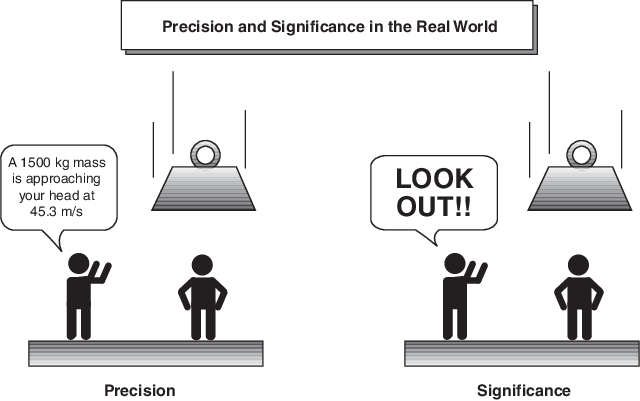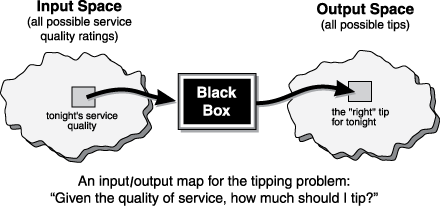## What Is Fuzzy Logic?

### Description of Fuzzy Logic

In recent years, the number and variety of applications of fuzzy logic have increased significantly. The applications range from consumer products such as cameras, camcorders, washing machines, and microwave ovens to industrial process control, medical instrumentation, decision-support systems, and portfolio selection.

To understand why use of fuzzy logic has grown, you must first understand what is meant by fuzzy logic.

Fuzzy logic has two different meanings. In a narrow sense, fuzzy logic is a logical system, which is an extension of multivalued logic. However, in a wider sense fuzzy logic (FL) is almost synonymous with the theory of fuzzy sets, a theory which relates to classes of objects with unsharp boundaries in which membership is a matter of degree. In this perspective, fuzzy logic in its narrow sense is a branch of FL. Even in its more narrow definition, fuzzy logic differs both in concept and substance from traditional multivalued logical systems.

In Fuzzy Logic Toolbox™ software, fuzzy logic should be interpreted as FL, that is, fuzzy logic in its wide sense. The basic ideas underlying FL are explained in Foundations of Fuzzy Logic. What might be added is that the basic concept underlying FL is that of a linguistic variable, that is, a variable whose values are words rather than numbers. In effect, much of FL may be viewed as a methodology for computing with words rather than numbers. Although words are inherently less precise than numbers, their use is closer to human intuition. Furthermore, computing with words exploits the tolerance for imprecision and thereby lowers the cost of solution.

Another basic concept in FL, which plays a central role in most of its applications, is that of a fuzzy if-then rule or, simply, fuzzy rule. Although rule-based systems have a long history of use in Artificial Intelligence (AI), what is missing in such systems is a mechanism for dealing with fuzzy consequents and fuzzy antecedents. In fuzzy logic, this mechanism is provided by the calculus of fuzzy rules. The calculus of fuzzy rules serves as a basis for what might be called the Fuzzy Dependency and Command Language (FDCL). Although FDCL is not used explicitly in the toolbox, it is effectively one of its principal constituents. In most of the applications of fuzzy logic, a fuzzy logic solution is, in reality, a translation of a human solution into FDCL.

A trend that is growing in visibility relates to the use of fuzzy logic in combination with neurocomputing and genetic algorithms. More generally, fuzzy logic, neurocomputing, and genetic algorithms may be viewed as the principal constituents of what might be called soft computing. Unlike the traditional, hard computing, soft computing accommodates the imprecision of the real world. The guiding principle of soft computing is: Exploit the tolerance for imprecision, uncertainty, and partial truth to achieve tractability, robustness, and low solution cost. In the future, soft computing could play an increasingly important role in the conception and design of systems whose MIQ (Machine IQ) is much higher than that of systems designed by conventional methods.

Among various combinations of methodologies in soft computing, the one that has highest visibility at this juncture is that of fuzzy logic and neurocomputing, leading to neuro-fuzzy systems. Within fuzzy logic, such systems play a particularly important role in the induction of rules from observations. An effective method developed by Dr. Roger Jang for this purpose is called ANFIS (Adaptive Neuro-Fuzzy Inference System). This method is an important component of the toolbox.

Fuzzy logic approximates human reasoning and does a good job of balancing the tradeoff between precision and significance. For instance, when warning someone of an object falling toward them, being precise about the exact mass and speed is not necessary.Fuzzy logic is a convenient way to map an input space to an output space. Mapping input to output is the starting point for everything. Consider the following examples:

• With information about how good your service was at a restaurant, a fuzzy logic system can tell you what the tip should be.

• With your specification of how hot you want the water, a fuzzy logic system can adjust the faucet valve to the right setting.

• With information about how far away the subject of your photograph is, a fuzzy logic system can focus the lens for you.

• With information about how fast the car is going and how hard the motor is working, a fuzzy logic system can shift gears for you.

A fuzzy system behaves like a black box that maps an input space to an output space. For example, you can map the input space of all possible restaurant service ratings to all possible tip values.Determining the appropriate amount of tip requires mapping inputs to the appropriate outputs. Between the input and the output, the preceding figure shows a black box that can contain any number of things: fuzzy systems, linear systems, expert systems, neural networks, differential equations, interpolated multidimensional lookup tables, or even a spiritual advisor, just to name a few of the possible options. Clearly the list could go on and on.

Of the dozens of ways to make the black box work, it turns out that fuzzy is often the very best way. Why should that be? As Lotfi Zadeh, who is considered to be the father of fuzzy logic, once remarked: "In almost every case you can build the same product without fuzzy logic, but fuzzy is faster and cheaper."

### Why Use Fuzzy Logic?

Here is a list of general observations about fuzzy logic:

• Fuzzy logic is conceptually easy to understand.

The mathematical concepts behind fuzzy reasoning are very simple. Fuzzy logic is a more intuitive approach without the far-reaching complexity.

• Fuzzy logic is flexible.

With any given system, it is easy to layer on more functionality without starting again from scratch.

• Fuzzy logic is tolerant of imprecise data.

Everything is imprecise if you look closely enough, but more than that, most things are imprecise even on careful inspection. Fuzzy reasoning builds this understanding into the process rather than tacking it onto the end.

• Fuzzy logic can model nonlinear functions of arbitrary complexity.

You can create a fuzzy system to match any set of input-output data. This process is made particularly easy by adaptive techniques like Adaptive Neuro-Fuzzy Inference Systems (ANFIS), which are available in Fuzzy Logic Toolbox software.

• Fuzzy logic can be built on top of the experience of experts.

In direct contrast to neural networks, which take training data and generate opaque, impenetrable models, fuzzy logic lets you rely on the experience of people who already understand your system.

• Fuzzy logic can be blended with conventional control techniques.

Fuzzy systems don't necessarily replace conventional control methods. In many cases fuzzy systems augment them and simplify their implementation.

• Fuzzy logic is based on natural language.

The basis for fuzzy logic is the basis for human communication. This observation underpins many of the other statements about fuzzy logic. Because fuzzy logic is built on the structures of qualitative description used in everyday language, fuzzy logic is easy to use.

The last statement is perhaps the most important one and deserves more discussion. Natural language, which is used by ordinary people on a daily basis, has been shaped by thousands of years of human history to be convenient and efficient. Sentences written in ordinary language represent a triumph of efficient communication.

### When Not to Use Fuzzy Logic

Fuzzy logic is not a cure-all. When should you not use fuzzy logic? The safest statement is the first one made in this introduction: fuzzy logic is a convenient way to map an input space to an output space. If you find it's not convenient, try something else. If a simpler solution already exists, use it. Fuzzy logic is the codification of common sense — use common sense when you implement it and you will probably make the right decision. Many controllers, for example, do a fine job without using fuzzy logic. However, if you take the time to become familiar with fuzzy logic, you'll see it can be a very powerful tool for dealing quickly and efficiently with imprecision and nonlinearity.

### What Can Fuzzy Logic Toolbox Software Do?

Using Fuzzy Logic Toolbox software, you can:

• Create and edit fuzzy inference systems using command-line functions or the Fuzzy Logic Designer app.

• Automatically generate fuzzy systems using clustering or adaptive neuro-fuzzy techniques.

• Automatically tune the parameters of a fuzzy logic system using optimization methods such as genetic algorithms and particle swarm optimization. For more information, see Tuning Fuzzy Inference Systems.

• Simulate your fuzzy system within a Simulink® model using the Fuzzy Logic Controller block.

• Automatically generate code for evaluating fuzzy inference systems. For more information, see Deploy Fuzzy Inference Systems.